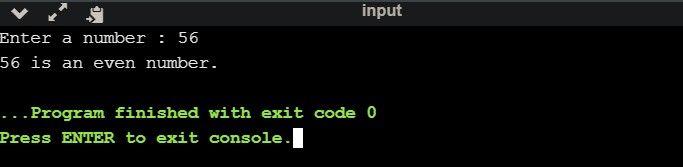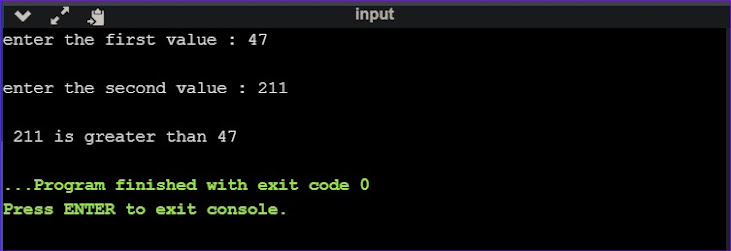What is If - Else ?

If-else statement in C++ is used to perform the operations specified in if portions are executed if and only if the given condition is true and it's based on some specific condition.

1. If statement :

If statements stands for modifying the flow of execution of a program. It evaluated the condition first before executing any statements inside the body of it.

Syntax :
if(condition)
{
// statement will be executed if the condition is true
}

Sample Program :
#include<iostream>
using namespace std;
int main ()
{
int x = 2;
// checking the boolean condition
if( x < 10 )
{
// if condition is true then it'll print the following
cout << "The value of x is smaller than 10";
}
else
{
// if condition is false then it'll print the following
cout << " x = " << x;
}
return 0;
}

Output :2. If-else statement :

It is an extended version of If statement. Here we use if statement for one condition and else statement to do something if the if condition is false.

Syntax :
if( expression)
{
statement;  /*if the expression is true then it will print this part.*/
} ;
else
{
statement;  //if the expression is false then it will print this part.
};

Sample Program :
#include<iostream>
using namespace std;
int main ()
{
int T;
cout << "Enter a number : ";
cin >> T;
if (T % 2 == 0)
{
cout << T <<" is an even number.";
}
else
{
cout << T <<" is an odd number.";
}
return 0;
}

Output :3. If else-If statement :

Deciding among multiple options we use if else-if statement or if else-if ladder. As one of the if condition is true then the associated statements with that if condition will be executed, and the rest of the ladder will be bypassed. And if none of the conditions get true then the last else statement will be executed.

Syntax :
if (expression)
{
statement;    // if the expression is true this part will be printed.
} ;
else if (another expression)
{
statement;    // if the another expression is satisfied print this part will be printed.
};
else
{
statement;    // if all the expression is false then this part will be printed.
};

Sample Program :
#include<iostream>
using namespace std;
int main()
{
int a, p;
cout<<"enter the first value : ";
cin >> a;
cout<<"\nenter the second value : ";
cin>>p;
if (a > p)
{
cout <<endl <<a <<" is greater than "<< p;
}
else if (p > a)
{
cout <<endl <<p <<" is greater than "<< a;
}
else
{
cout <<endl <<"both " <<a <<" and " << p <<" are equal";
}
return 0;
}

Output :4. Nested if statement :

Nested if statement is nothing but an if statement inside another if statement.

Syntax :
if( main expression )
{
//will be executed when condition 1 is true.
if(nested expression )
{
// will be executed when condition 2 is true.
}
}

Sample Program :
#include<iostream>
using namespace std;
int main()
{
int mark;
cout <<"enter the marks to check : ";
cin >>mark;
if (mark >= 50)
{
// Nested if
if (mark == 100)
{
// This will be executed when above statement is true.
cout <<"Perfect!!";
}
cout<<" You passed.";
}
else
{
cout <<"You failed. Try harder";
}
}

Output :